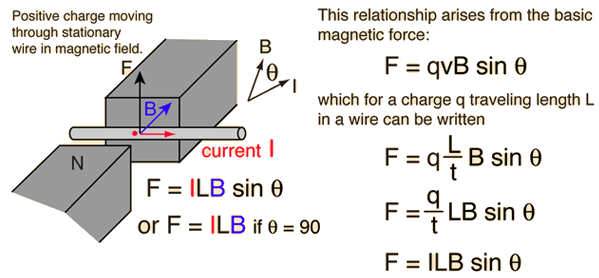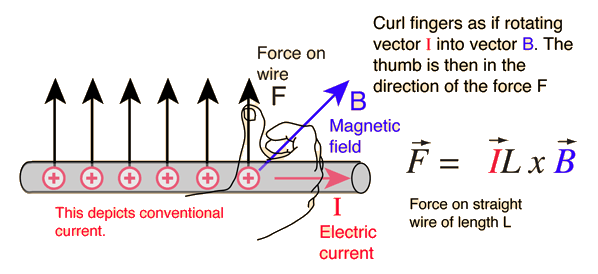# Magnetic Force on a Current-Carrying WireThe magnetic force on a current-carrying wire is perpendicular to both the wire and the magnetic field with direction given by the right hand rule.If the current is perpendicular to the magnetic field then the force is given by the simple product:

## Force = Current x Length x B-field

For current I = A = x 10^ A
and length L = x 10^ m
positioned perpendicular to a magnetic field B = Tesla = Gauss
the force is F = x 10^ N.
If the angle between the current and magnetic field is degrees
the force is F = x 10^ N.

Data may be entered in any of the fields. Whey you have finished entering data, click on the quantity you wish to calculate in the active formula above. The quantities will not be forced to be consistent until you click on a choice. Default values will be entered for unspecified parameters, but all values may be changed.

 Magnetic interactions with charge
 Magnetic force applications
Index

Electromagnetic force

Magnetic field concepts

 HyperPhysics***** Electricity and Magnetism R Nave
Go Back